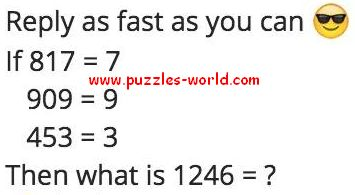# If 817 = 7 909=9 453=3 the what is 1246 = ?Reply as fast as you can
If
817 = 7
909 = 9
453 = 3
Then what is 1246 = ?